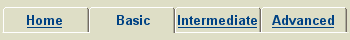Classpad Help Series - BasicClick a title to play a short movie in the right hand pane. Choose PDF to open a printable help sheet in a new window.Starting Out In Main 001 Setting Up 1 (Handheld video) PDF 002 Setting Up 2 PDF 005 Exploring The Keyboard PDF 006 Basic Editing in Main PDF 007 Simple Calculations in Main PDF 010 Numeric Calculations PDF 011 Simplifying and Expanding PDF 012 Factorising PDF 013 Simplifying Algebraic Fractions PDF 014 HCF and LCM PDFSolving Equations 200 Three Actions Before Solving PDF 201 Solving Equations Step By Step PDF 202 Solving Equations With Solve PDF 203 Solving Linear Inequalities PDF 204 Solving Quadratic Equations PDF 205 Solving Simultaneous Linear Eqns 1 PDF 206 Solving Simultaneous Linear Eqns 2 PDF 207 Solving Simultaneous Linear Eqns 3 PDF 208 Rearranging Equations PDFGraph and Table 300 Graph and Table Settings PDF 301 Graph a Linear Function 1 (Graph) PDF 302 Graph a Linear Function 2 (Main) PDF 303 Graph a Quadratic Function PDF 304 Graph an Exponential Function PDF 305 Graph Simultaneous Equations 1 (Linear) PDF 306 Graph Simultaneous Equations 2 (Quadratic) PDF 307 Simultaneous Equations In Main PDFStatistics and Lists 400 Statistics: Basic Settings and Actions PDF 401 Statistical Calcs: From a List PDF 402 Statistical Calcs: Frequency Table PDF 403 Create a Boxplot from a List PDF 404 Create a Boxplot from a Frequency Table PDF 405 Create Multiple Boxplots PDF 406 Create a Histogram from a List PDF 407 Create a Histogram from a Frequency Table PDF 410 Statistical Calcs: Two Variables PDF 411 Scatterplot and Regression PDF 412 xyLine Time Series PDFSpreadsheets 500 Spreadsheet Basics PDF 501 Arithmetic Sequence PDF 502 Fibonacci Sequence PDF 503 Geometric Sequence PDF 520 Pie Chart PDFeActivities 601 Storing Formulae In eActivities PDFGeometry 700 Geometry - Basic Settings PDF 701 Geometry - Line Segment PDF 702 Geometry - Line Segment A To B PDF 703 Geometry - Line Segment Midpoint PDF 712 Geometry - Triangle PDF 713 Geometry - Right Triangle PDF 714 Geometry - Solve Triangle 1 PDF 715 Geometry - Solve Triangle 2 PDF 716 Geometry - Solve Triangle 3 PDFSequence 800 Sequence Basics PDF 801 Sequence Explicity Defined PDF 802 Simple Recursive Sequences PDF 803 Fibonacci Type Sequences PDFOther Applications 901 Algy - An Introduction PDF 902 Algy - Squaring An Expression PDF 912 NumSolve Tips PDF 913 Built-In Probability Simulation Tool PDF 921 Financial Simple Interest PDF 922 Financial Compound Interest PDF 923 Financial Loan Repayment PDFComplete Collection The complete collection of help sheets can be downloaded as a single pdf document CP000 Classpad Help Series.pdf.